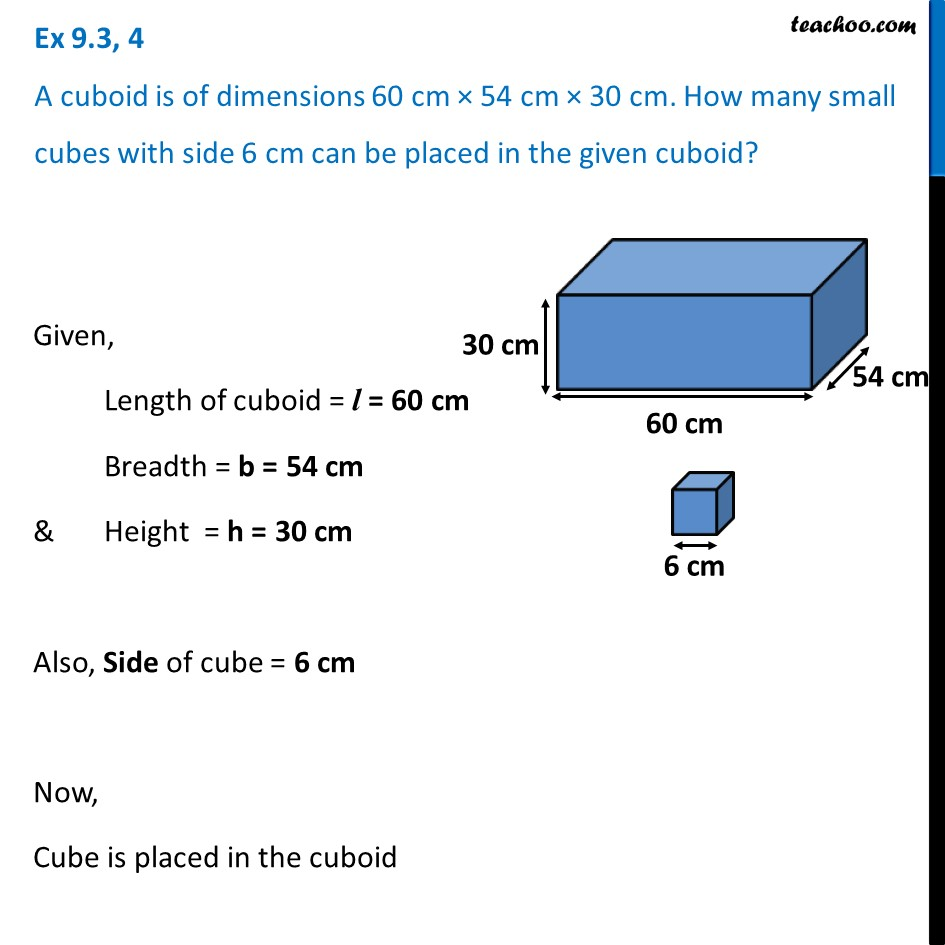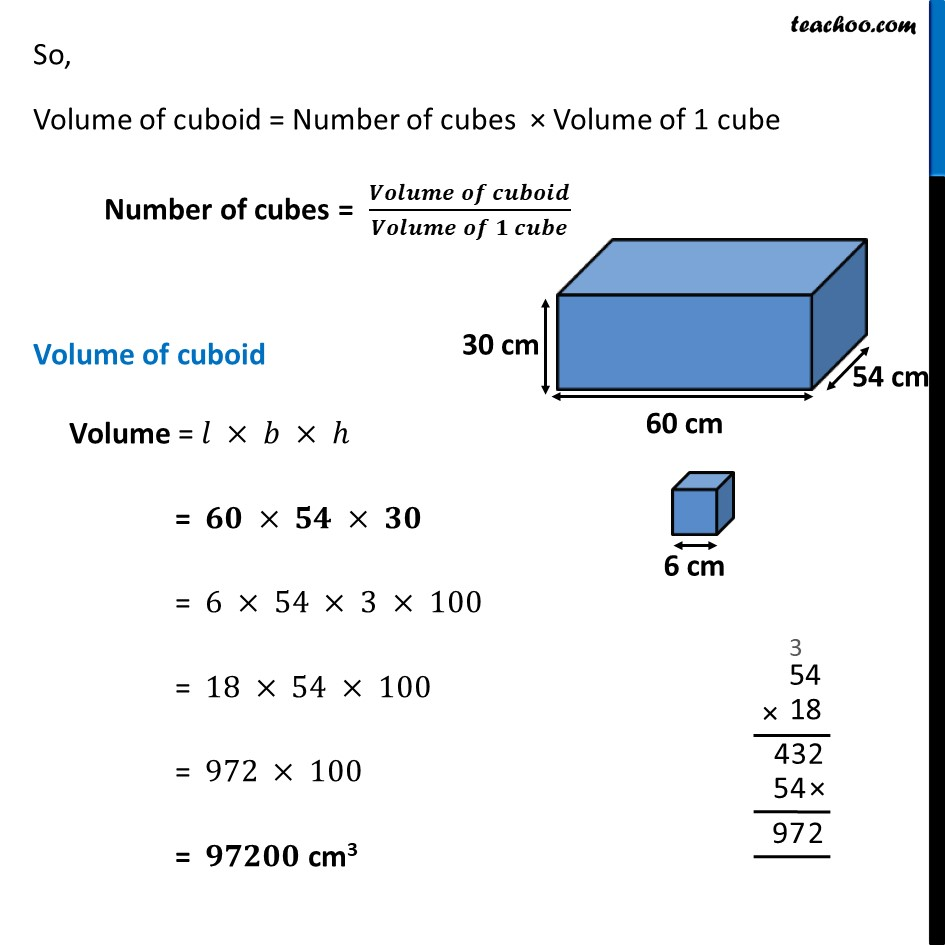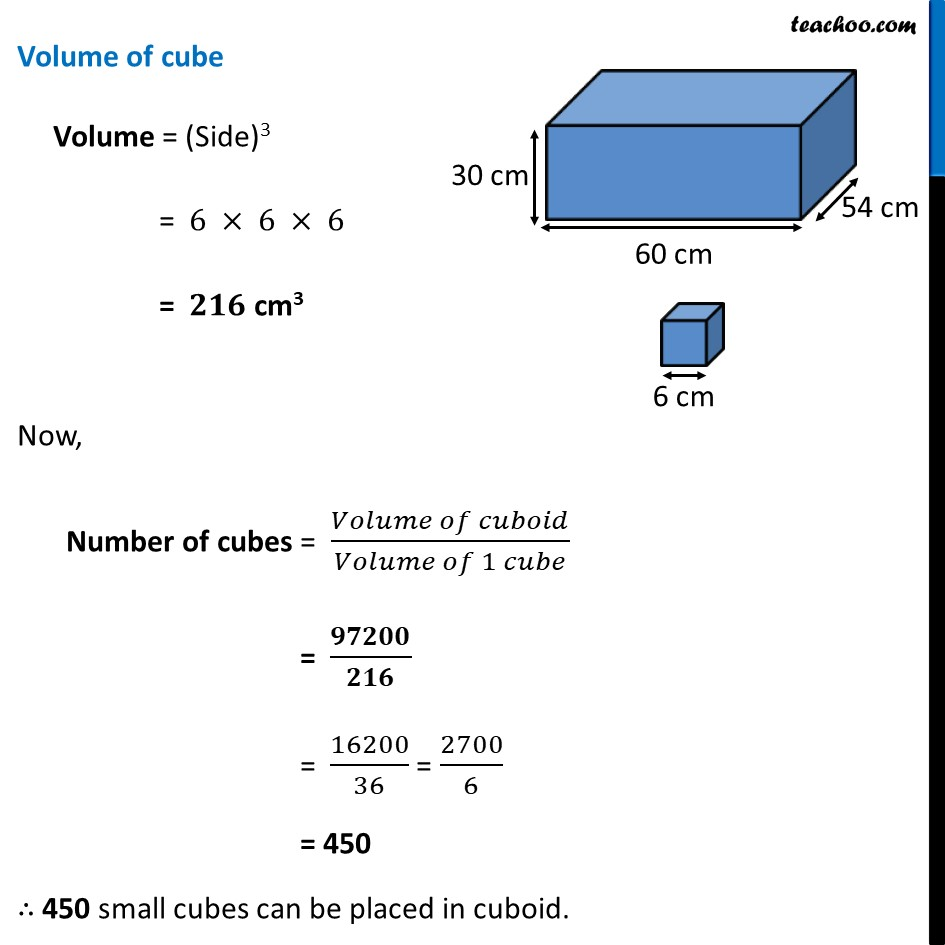Ex 9.3

Chapter 9 Class 8 Mensuration
Serial order wiseLearn in your speed, with individual attention - Teachoo Maths 1-on-1 Class

### Transcript

Ex 9.3, 4 A cuboid is of dimensions 60 cm × 54 cm × 30 cm. How many small cubes with side 6 cm can be placed in the given cuboid?Given, Length of cuboid = l = 60 cm Breadth = b = 54 cm & Height = h = 30 cm Also, Side of cube = 6 cm Now, Cube is placed in the cuboid So, Volume of cuboid = Number of cubes × Volume of 1 cube Number of cubes = (𝑽𝒐𝒍𝒖𝒎𝒆 𝒐𝒇 𝒄𝒖𝒃𝒐𝒊𝒅)/(𝑽𝒐𝒍𝒖𝒎𝒆 𝒐𝒇 𝟏 𝒄𝒖𝒃𝒆) Volume of cuboid Volume = 𝑙 × 𝑏 × ℎ = 𝟔𝟎 × 𝟓𝟒 × 𝟑𝟎 = 6 × 54 × 3 × 100 = 18 × 54 × 100 = 972 × 100 = 𝟗𝟕𝟐𝟎𝟎 cm3 Volume of cube Volume = (Side)3 = 6 × 6 × 6 = 𝟐𝟏𝟔 cm3 Now, Number of cubes = (𝑉𝑜𝑙𝑢𝑚𝑒 𝑜𝑓 𝑐𝑢𝑏𝑜𝑖𝑑)/(𝑉𝑜𝑙𝑢𝑚𝑒 𝑜𝑓 1 𝑐𝑢𝑏𝑒) = 𝟗𝟕𝟐𝟎𝟎/𝟐𝟏𝟔 = 16200/36 = 2700/6 = 450 ∴ 450 small cubes can be placed in cuboid.